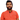# Learn Pain LessHomeOur TeamContact
Python
How to Rotate and Scale a Vector in PythonPawneshwer Gupta
March 06, 2023
1 min

01
Rotation of a Vector in Python
02
Scaling of a Vector in Python
03
Combining Rotation and Scaling
04
ConclusionRotating and scaling vectors are common operations in computer graphics and machine learning applications. Python provides a rich library of tools for performing such operations. In this article, we will explore how to rotate and scale a vector in Python.

## .css-1qh5hbx{box-sizing:border-box;margin:0;min-width:0;display:block;color:var(--theme-ui-colors-heading,#2d3748);font-weight:bold;-webkit-text-decoration:none;text-decoration:none;margin-bottom:1rem;font-size:1.5rem;position:relative;}Rotation of a Vector in Python

A vector in two-dimensional space can be represented by a tuple or list of two numbers. The first number represents the horizontal component, and the second represents the vector’s vertical component. We can rotate a vector by a certain angle using the following formula:

```.css-1baulvz{display:inline-block;}import math
def rotate_vector(vector, angle):    x = vector * math.cos(angle) - vector * math.sin(angle)    y = vector * math.sin(angle) + vector * math.cos(angle)    return [x, y]```

Here, `vector` is the vector to be rotated, and `angle` is the angle (in radians) we want to rotate the vector. The `math.cos` and `math.sin` functions are used to compute the cosine and sine of the angle, respectively. The rotated vector is returned as a list of two numbers.

## Scaling of a Vector in Python

We can scale a vector by multiplying its horizontal and vertical components by a scaling factor. This can be achieved using the following code:

`def scale_vector(vector, scale):    x = vector * scale    y = vector * scale    return [x, y]`

Here, `vector` is the vector to be scaled, and `scale` is the scaling factor. The scaled vector is returned as a list of two numbers.

## Combining Rotation and Scaling

We can combine rotation and scaling operations to achieve more complex transformations. For example, suppose we want to rotate a vector by an angle of 45 degrees and then scale it by a factor of 2. We can achieve this using the following code:

```vector = [1, 1]angle = math.radians(45)scale = 2
# Rotate the vectorvector = rotate_vector(vector, angle)
# Scale the vectorvector = scale_vector(vector, scale)
print(vector)  # Output: [1.414213562373095, 1.414213562373095]```

Here, we first define the vector to be transformed (`vector`), the angle by which we want to rotate the vector (`angle`), and the scaling factor (`scale`). We then rotate the vector using the `rotate_vector` function, and scale the resulting vector using the `scale_vector` function. The final transformed vector is printed to the console.

## Conclusion

In this article, we have explored how to rotate and scale a vector in Python. These operations are useful in various applications, including computer graphics, machine learning, and physics simulations. Using the code snippets provided in this article, you can easily incorporate these operations into your Python projects.

We'll send you the best of our blog just once a month. We promise.

Vector

## Software Developer

Pawneshwer Gupta works as a software engineer who is enthusiastic in creating efficient and innovative software solutions.

Python
Flutter
Laravel
NodeJS

## Related PostsUnderstanding Python deque (Double-Ended Queue)
August 23, 2023
2 min